Chapter 3

In our electron model, we no longer regard the electron as a point-like particle. Similar to the electron’s electric charge, which has continuum distribution inside of the electron, we make one of our basic assumptions here that the magnetic charge has continuum distribution inside of the electron. The distribution equation is as follows: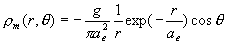(3.1)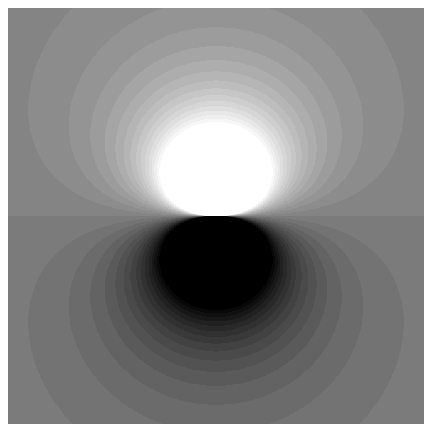By integrating the magnetic charge density equation (3.1), we can get the volume magnetic charge: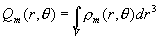(3.2)

In which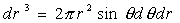thus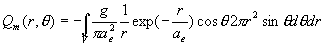(3.3)

Therefore,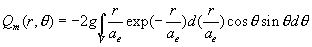(3.4)

Thus: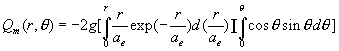(3.5)

Consequently,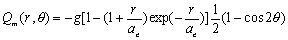(3.6)

Equation (3.6) is the electron magnetic charge space distribution equation.

From the above equation (3.6), the radial part of the distribution is a kind of cumulative gamma distribution function *[B].

From equation (
3.6), we find out that:

When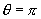, Thus: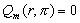(3.7)

From equation (3.7), we learn that within the sphere of any radius r, the magnetic charge total is always zero.

We find out that inside of the electron, within the sphere of any radius r, the magnetic charge as a whole is always zero, even though magnetic charge distribution exists inside of the electron.

When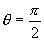, thus:(3.8)

The above equation (3.8) is the electron north pole area magnetic charge distribution within the sphere of radius r, from which we can see the magnetic charge distribution is also the cumulative gamma distribution [B].

When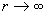thus: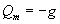(3.9)

Which shows us that the electron has one unit negative magnetic charge ‘g’ in the whole north pole area (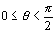).
Inside the electron, the whole area of the north pole, has one unit negative magnetic charge ‘g’.

As we know, the electron has total zero magnetic charge, so the electron whole south pole area (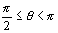) has one unit positive magnetic charge ‘g.

Inside the electron, the whole area of south pole has one unit positive magnetic charge ‘g’.

Reference

Quantised singularities in the electromagnetic field
PAM. Dirac
Proc. Roy. Soc. London A 133, 60, 1931

The theory of magnetic monopoles
PAM. Dirac
Phys. Rev. 74, 817-830 (1948)

P V Panat

Eur. J. Phys. 24 111-114 (2003)

W. B. Zeleny

Am. J. Phys. 59, 412 (1991)

Am. J. Phys. 59, 410 (1991)

K. R. Brownstein

Am. J. Phys. 57, 420 (1989)